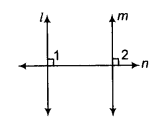# Mukesh asked the teacher whether the two lines which are perpendicular to the same line,

Mukesh asked the teacher whether the two lines which are perpendicular to the same line, are perpendicular to each other or not. His teacher replied that yes, they will be perpendicular to each other giving the reason that two lines parallel to the same line are parallel to each other. His classmate Seema told him that he is wrong and explain him the correct statement. Mukesh thanked Seema for this.
(i) Write the solution given by Seema.
(ii) Identify the value depicted from this action.

(i) Let l and m be two lines perpendicular to the same line n as shown below.Here, ∠1 = 90° and ∠2 = 90° [given]
∴ ∠1 = ∠2
Also, Z1 and Z2 are corresponding angles for lines l and m intersected by the transversal line n.
∴ l||m
Hence, lines l and m are parallel to each other.
(ii) The value has been shown in this question is cooperative learning among students.